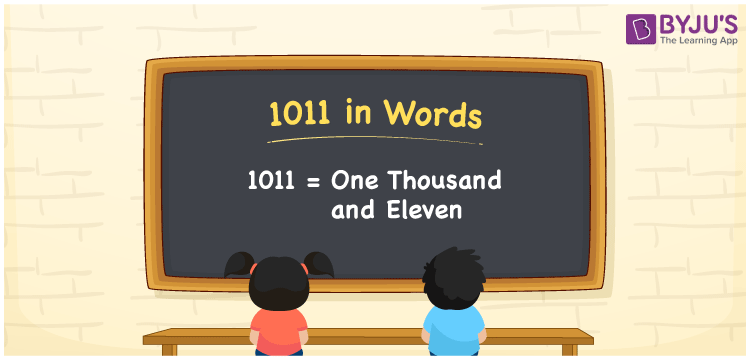# 1011 in Words

We can write 1011 in words as One thousand and eleven. We can effortlessly convert 1011 into words using a place value chart. Also, we know that the word form of the number 1011 is used to express or spell that number. Suppose you spent Rs. 1011 to buy flowers, you could say, “I spent One thousand and eleven rupees for flowers”. In this article, you will learn how to write the cardinal number 1011 in words.

 1011 in words One thousand and eleven One thousand and eleven in Numbers 1011

## 1011 in English Words

We generally write numbers in words using the English alphabet. So, we can read 1011 in English as “One thousand and eleven”.## How to Write 1011 in Words?

In this section, you will learn how to write the number 1011 in words using a place value chart with four columns as shown below.

 Thousands Hundreds Tens Ones 1 0 1 1

Here, ones = 1, tens = 1, hundreds = 0, thousands = 1

Let us expand these digits based on the place values.

1 × Thousand + 0 × Hundred + 1 × Ten + 1 × One

= 1 × 1000 + 0 × 100 + 1 × 10 + 1 × 1

= 1000 + 10 + 1

= One thousand + Ten + One

= One thousand and eleven

Therefore, 1011 in words = One thousand and eleven.

As we know, 1011 is a natural number that precedes 1012 and succeeds 1010.

1011 in words – One thousand and eleven

Is 1011 an odd number? – Yes

Is 1011 an even number? – No

Is 1011 a perfect square number? – No

Is 1011 a perfect cube number? – No

Is 1011 a prime number? – No

Is 1011 a composite number? – Yes

## Frequently Asked Questions on 1011 in Words

Q1

### How do you write 1011 in words?

We can write 1011 in words as One thousand and eleven.
Q2

### What is the spelling of 1011?

The spelling of 1011 in English is One thousand and eleven. Therefore, the number 1011 is spelt as One thousand and eleven.
Q3

### How to write Rs. 1011 in words on a cheque?

On a cheque, we generally express Rs. 1011 in words as “One thousand and eleven rupees only”.